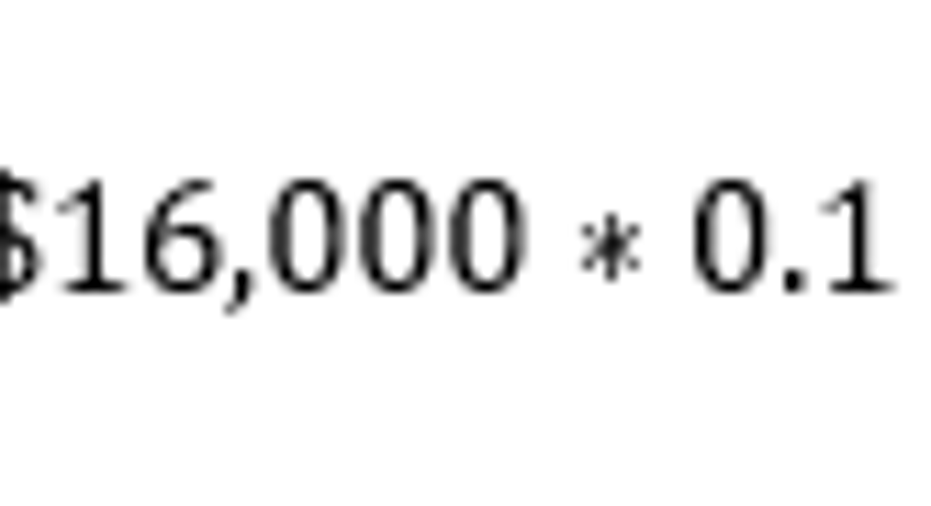# Difference Between Straight-Line Depreciation and Declining Balance DepreciationWhen a business acquires an asset to be used in its operations, the cost of the asset is generally not expensed all at once. Rather, the cost is depreciated over a period of time that depends on the useful life of the asset.

There are two main methods of calculating depreciation, the straight-line method and the declining balance method. Here's the difference between the two, and when each method might be useful.

Straight-line depreciationThe straight-line method of depreciation is the easiest to calculate, and consists of depreciating the value of an asset in equal installments over the cost of its useful life. In order to calculate straight-line depreciation, you'll need the following information:

• The asset's initial cost
• The salvage value (It's estimated value at the end of its useful life)
• The useful lifespan, in years

First, divide the number one by the useful life to get the annual depreciation rate:

Next, subtract the salvage value from the initial cost to find the depreciable cost:

Finally, multiply the annual depreciation rate by the depreciable cost to arrive at the annual straight-line depreciation amount.

For example, let's say that your business buys a piece of equipment for \$20,000 with a useful life of 10 years and an expected salvage value of \$4,000. We can calculate the annual depreciation rate to be 0.1, or 10%, and the depreciable cost to be the difference between \$20,000 and \$4,000, or \$16,000. Therefore, the annual depreciation is:

Declining balance depreciationThe declining balance method calculates more depreciation expense initially, and uses a percentage of the asset's current book value, as opposed to its initial cost. So, the amount of depreciation declines over time, and continues until the salvage value is reached.

For example, let's say that you buy new computers for your business at an initial cost of \$12,000, and you depreciate their value at 25% per year. If we estimate the salvage value at \$3,000, this is a total depreciable cost of \$10,000.

Year

Book value

Depreciation

Total depreciation

1

\$12,000

\$3,000

\$3,000

2

\$9,000

\$2,250

\$5,250

3

\$6,750

\$1,687

\$6,937

4

\$5,063

\$1,266

\$8,203

5

\$3,797

\$797

\$9,000

Note: Accumulated depreciation figures may be slightly off due to rounding

In the last line of the chart, notice that 25% of \$3,797 is \$949, not the \$797 that's listed. However, the total depreciation allowed is equal to the initial cost minus the salvage value, which is \$9,000. At the point where this amount is reached, no further depreciation is allowed.

Benefits of each methodThere are good reasons for using both of these methods, and the right one depends on the asset type in question. The straight-line depreciation method is the easiest to use, so it makes for simplified accounting calculations. On the other hand, the declining balance method often provides a more accurate accounting of an asset's value. For instance, if you buy a new computer or smartphones for your employees, these types of assets naturally lose more value early in their life than they do later on. Other assets lose their value in a steady manner (furniture or real estate are good examples), so it makes more sense to use straight-line depreciation in these cases.

In a nutshell, the depreciation method used depends on the nature of the assets in question, as well as the company's preference.

This article is part of The Motley Fool's Knowledge Center, which was created based on the collected wisdom of a fantastic community of investors. We'd love to hear your questions, thoughts, and opinions on the Knowledge Center in general or this page in particular. Your input will help us help the world invest, better! Email us atknowledgecenter@fool.com. Thanks -- and Fool on!

The article Difference Between Straight-Line Depreciation and Declining Balance Depreciation originally appeared on Fool.com.

Try any of our Foolish newsletter services free for 30 days. We Fools may not all hold the same opinions, but we all believe that considering a diverse range of insights makes us better investors. The Motley Fool has a disclosure policy.